# Bond Valuation Bond and Bond Valuation A bond

• Slides: 30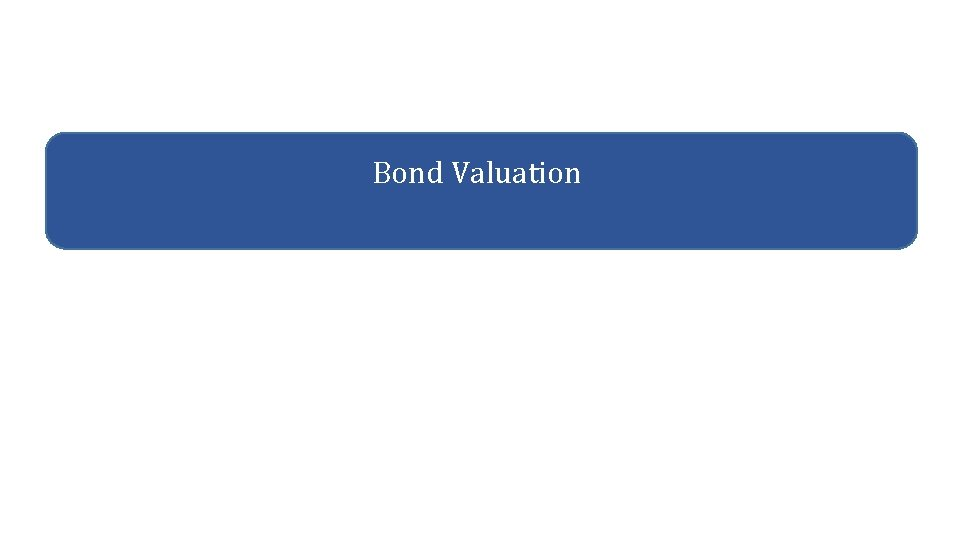Bond ValuationBond and Bond Valuation • A bond is a legally binding agreement between a borrower and a lender that specifies the: • • Par (face) value Coupon rate Coupon payment Maturity Date • The yield to maturity is the required market interest rate on the bond. Bond Valuation 2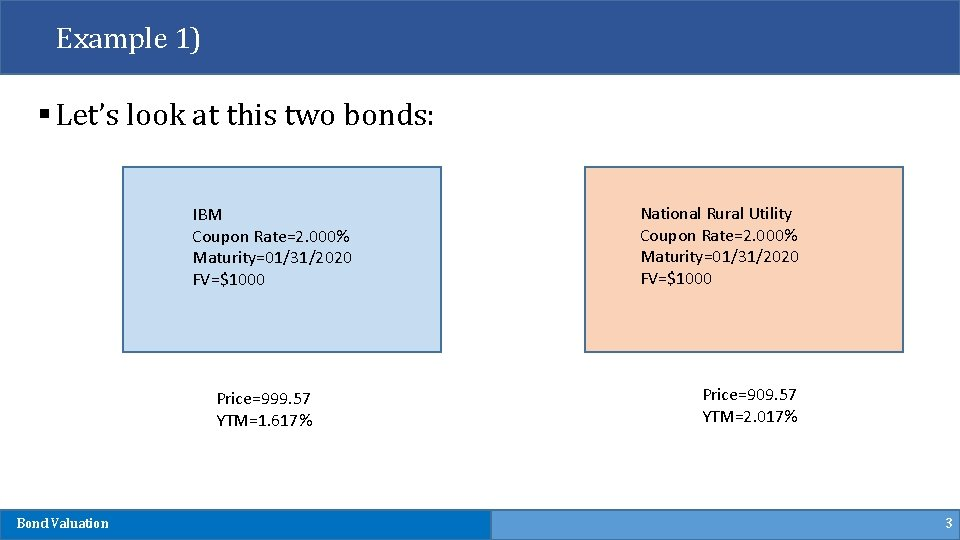Example 1) § Let’s look at this two bonds: IBM Coupon Rate=2. 000% Maturity=01/31/2020 FV=\$1000 Price=999. 57 YTM=1. 617% Bond Valuation National Rural Utility Coupon Rate=2. 000% Maturity=01/31/2020 FV=\$1000 Price=909. 57 YTM=2. 017% 3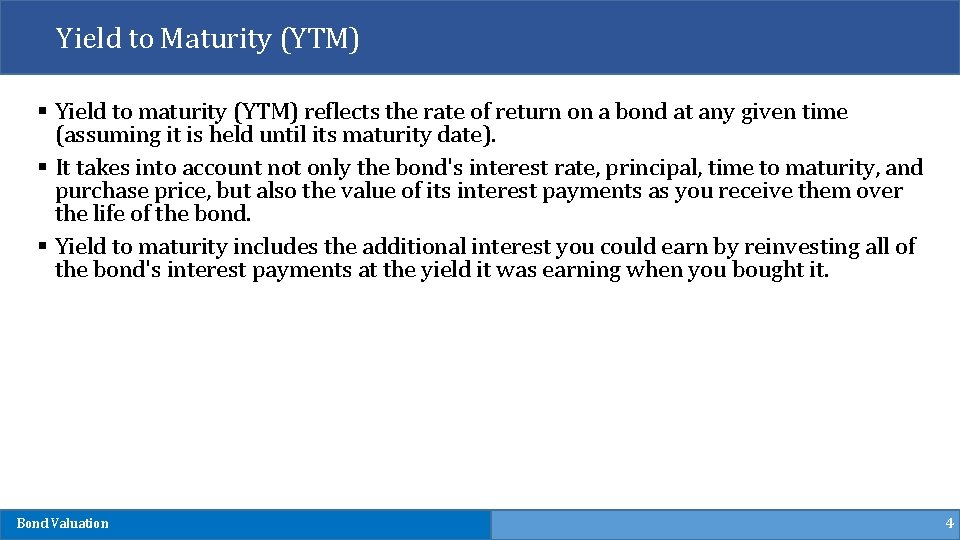Yield to Maturity (YTM) § Yield to maturity (YTM) reflects the rate of return on a bond at any given time (assuming it is held until its maturity date). § It takes into account not only the bond's interest rate, principal, time to maturity, and purchase price, but also the value of its interest payments as you receive them over the life of the bond. § Yield to maturity includes the additional interest you could earn by reinvesting all of the bond's interest payments at the yield it was earning when you bought it. Bond Valuation 4Why is YTM important? § Yield to maturity lets you accurately compare bonds with different maturities and coupon rates. § It's particularly helpful when you are comparing older bonds being sold in the secondary market that are priced at a discount or at a premium rather than face value. § It's also especially important when looking at a zero-coupon bond, which typically sells at a deep discount to its face value but makes no periodic interest payments. § Because you receive all of a zero's return at maturity, when its principal is repaid, any yield quoted for a zero-coupon bond is always a yield to maturity. Bond Valuation 5Bond Valuation • Primary Principle: • Value of financial securities = PV of expected future cash flows • Bond value is, therefore, determined by the present value of the coupon payments and par value. • Interest rates are inversely related to present (i. e. , bond) values. Bond Valuation 6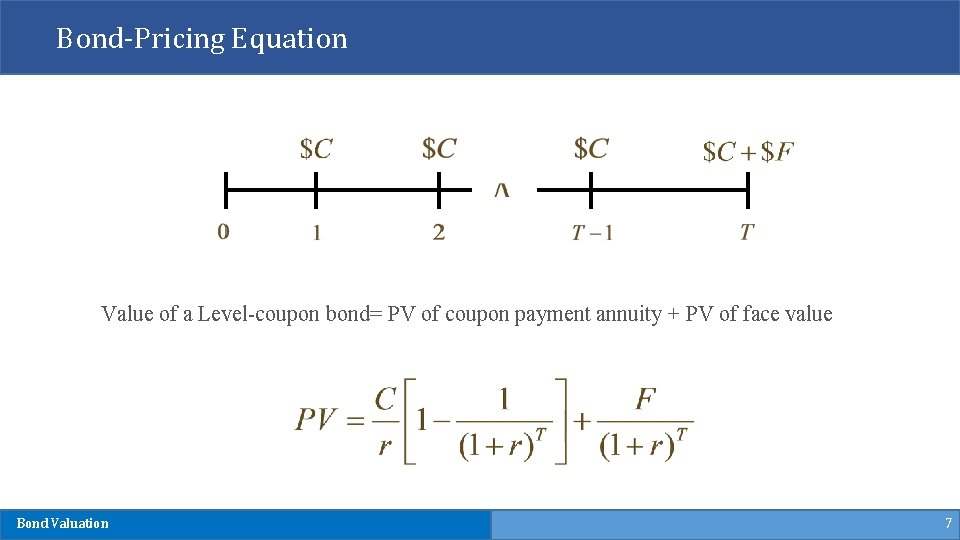Bond-Pricing Equation Value of a Level-coupon bond= PV of coupon payment annuity + PV of face value Bond Valuation 7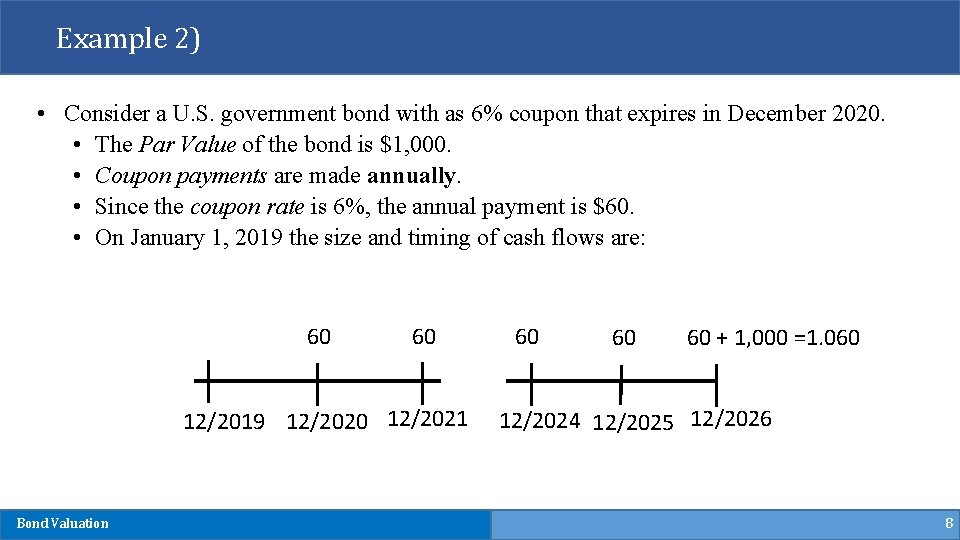Example 2) • Consider a U. S. government bond with as 6% coupon that expires in December 2020. • The Par Value of the bond is \$1, 000. • Coupon payments are made annually. • Since the coupon rate is 6%, the annual payment is \$60. • On January 1, 2019 the size and timing of cash flows are: 60 60 12/2019 12/2020 12/2021 Bond Valuation 60 60 60 + 1, 000 =1. 060 12/2024 12/2025 12/2026 8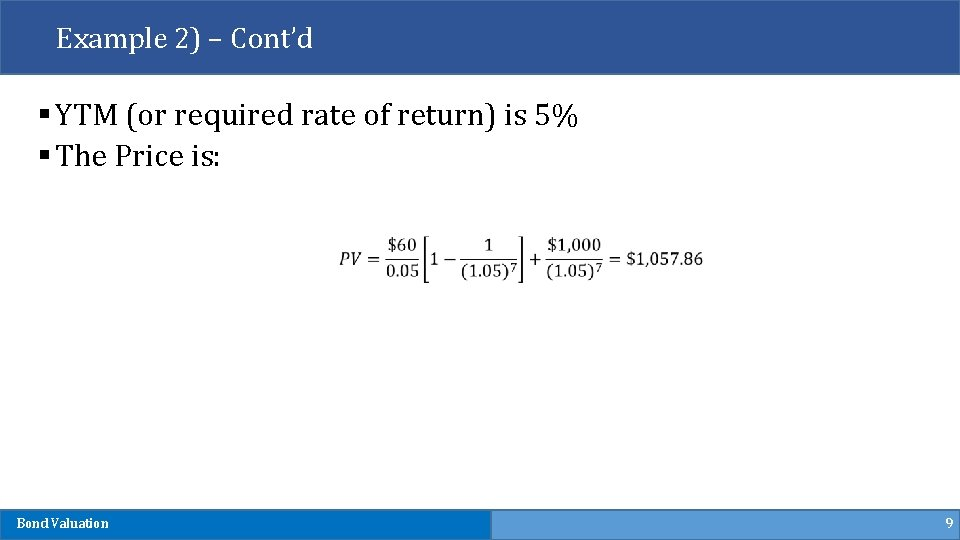Example 2) – Cont’d § YTM (or required rate of return) is 5% § The Price is: Bond Valuation 9Example 2) – Cont’d N = 7 I/YR = 5 PV = ? PMT = 60 FV Bond Valuation = 1057. 86 = 1000 10Example 3) § What if payments made semi-annually? N = 7 x 2=14 I/YR = 5/2=2. 5 PV = ? PMT = 60/2=30 FV Bond Valuation = 1058. 45 = 1000 11Example 4) § What if YTM (required return) increases to 10%? N I/YR = 10 PV = ? PMT = 60 FV Bond Valuation = 7 = 805. 26 = 1000 12Example 4) – Cont’d § Price sensitivity to interest rate change? YTM=5% YTM=10% Price=1, 057. 86 Price=805. 26 Bond Valuation 13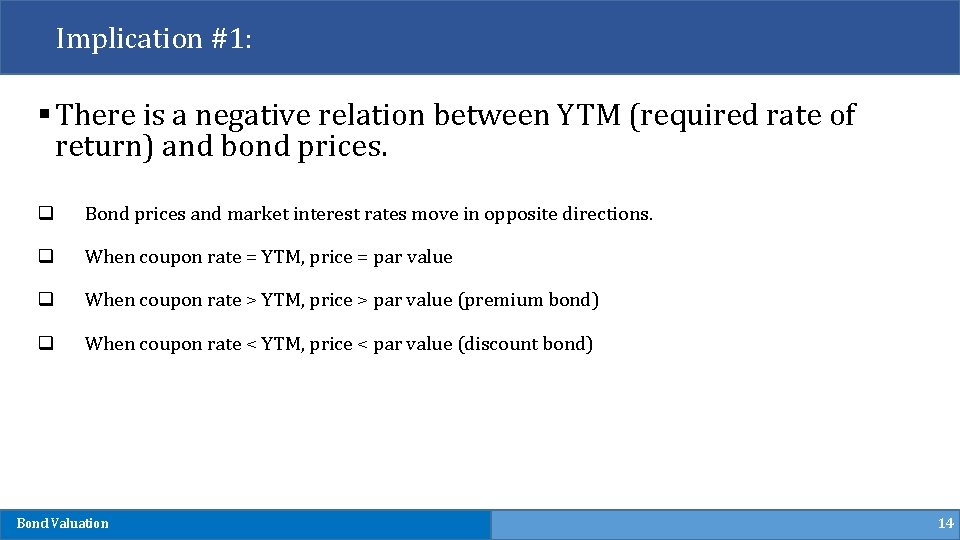Implication #1: § There is a negative relation between YTM (required rate of return) and bond prices. q Bond prices and market interest rates move in opposite directions. q When coupon rate = YTM, price = par value q When coupon rate > YTM, price > par value (premium bond) q When coupon rate < YTM, price < par value (discount bond) Bond Valuation 14Implication #1 When the YTM < coupon, the bond trades at a premium. Bond Value 1300 1200 When the YTM = coupon, the bond trades at par. 1100 1000 When the YTM > coupon, the bond trades at a discount. 800 0 Bond Valuation 0. 01 0. 02 0. 03 0. 04 0. 05 0. 06 6% 0. 07 0. 08 0. 09 0. 1 Discount Rate or YTM 15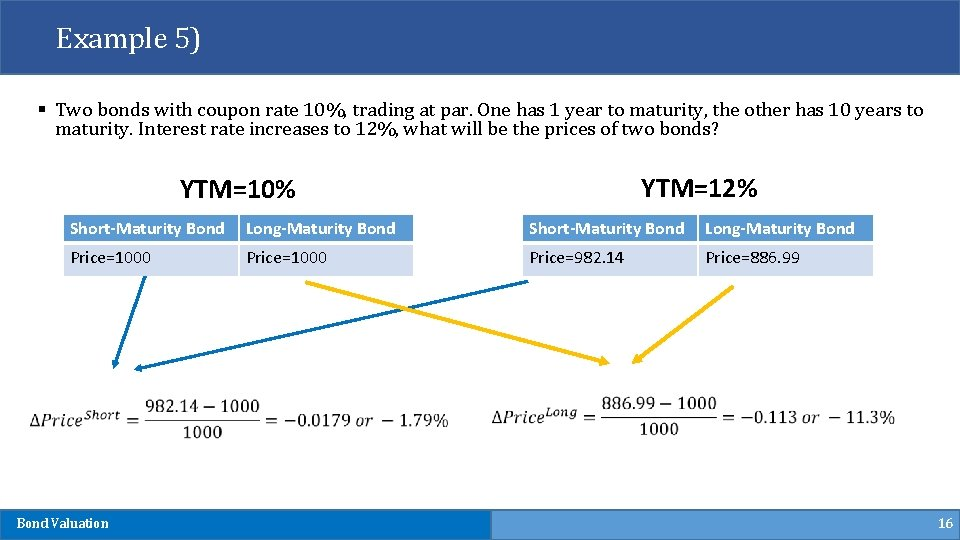Example 5) § Two bonds with coupon rate 10%, trading at par. One has 1 year to maturity, the other has 10 years to maturity. Interest rate increases to 12%, what will be the prices of two bonds? YTM=12% YTM=10% Short-Maturity Bond Long-Maturity Bond Price=1000 Price=982. 14 Price=886. 99 Bond Valuation 16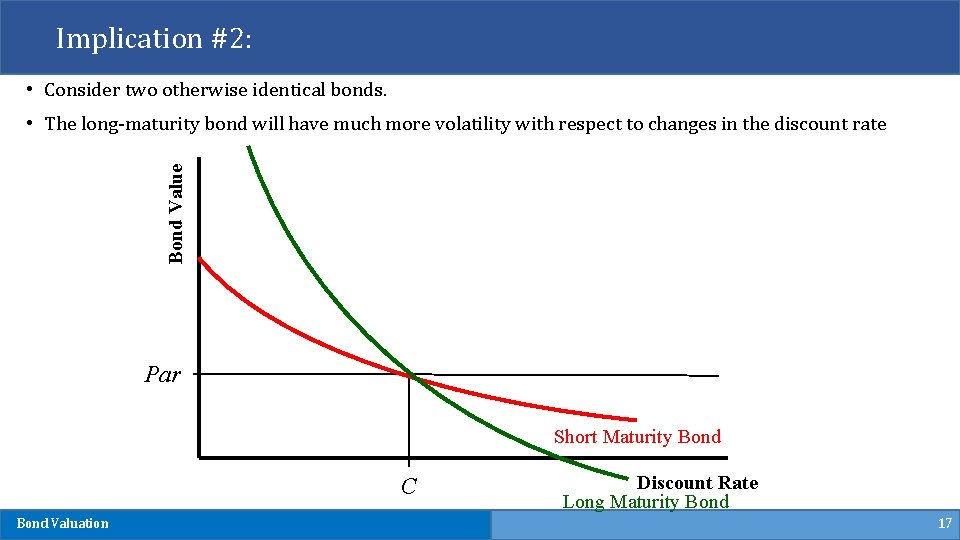Implication #2: • Consider two otherwise identical bonds. Bond Value • The long-maturity bond will have much more volatility with respect to changes in the discount rate Par Short Maturity Bond C Bond Valuation Discount Rate Long Maturity Bond 17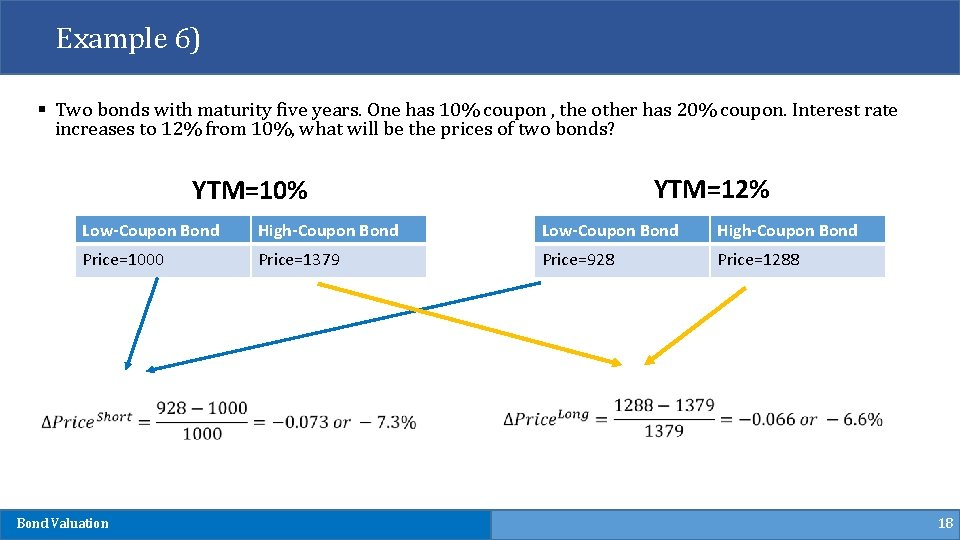Example 6) § Two bonds with maturity five years. One has 10% coupon , the other has 20% coupon. Interest rate increases to 12% from 10%, what will be the prices of two bonds? YTM=12% YTM=10% Low-Coupon Bond High-Coupon Bond Price=1000 Price=1379 Price=928 Price=1288 Bond Valuation 18Implication #3: • Consider two otherwise identical bonds. Bond Value • The low-coupon bond will have much more volatility with respect to changes in the discount rate. Par High Coupon Bond C Bond Valuation Low Coupon Bond Discount Rate 19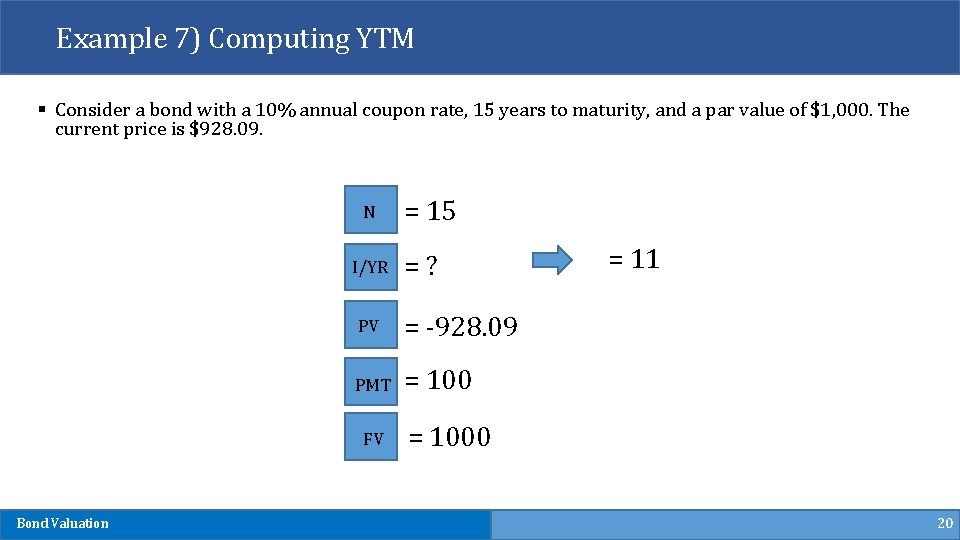Example 7) Computing YTM § Consider a bond with a 10% annual coupon rate, 15 years to maturity, and a par value of \$1, 000. The current price is \$928. 09. N I/YR = ? PV = -928. 09 PMT = 100 FV Bond Valuation = 15 = 11 = 1000 20Example 8) § Suppose a bond with a 10% coupon rate and semiannual coupons has a face value of \$1, 000, 20 years to maturity, and is selling for \$1, 197. 93. N I/YR = ? PV = -1, 197. 93 PMT = 100/2=50 FV Bond Valuation = 20 x 2=40 Is this YTM? = 4 Semi -Annual = 4 x 2=8 Annually = 1000 21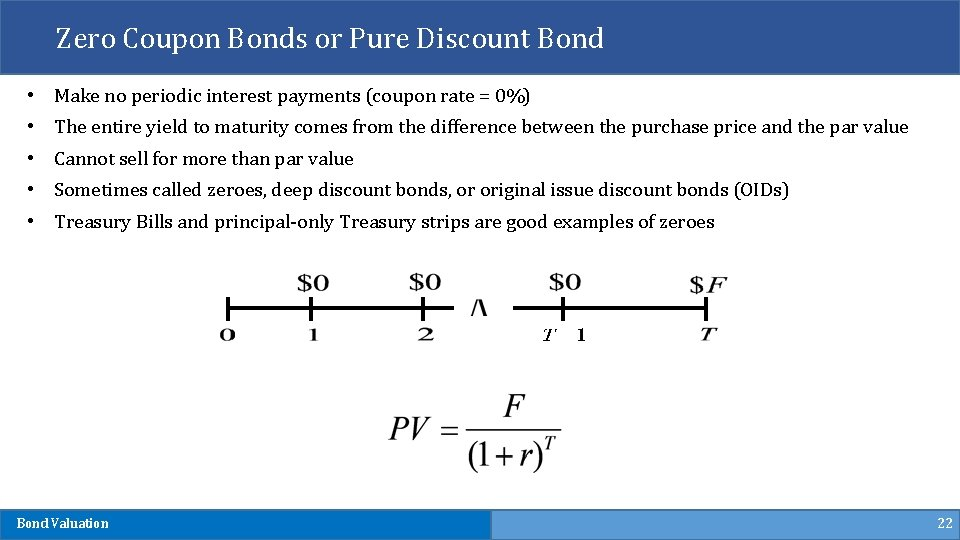Zero Coupon Bonds or Pure Discount Bond • Make no periodic interest payments (coupon rate = 0%) • The entire yield to maturity comes from the difference between the purchase price and the par value • Cannot sell for more than par value • Sometimes called zeroes, deep discount bonds, or original issue discount bonds (OIDs) • Treasury Bills and principal-only Treasury strips are good examples of zeroes Bond Valuation 22Example 8) § Find the value of a 15 -year zero-coupon bond with a \$1, 000 par value and a YTM of 12%. N = 15 I/YR = 12 PV = ? PMT = 0 FV Bond Valuation = 174. 11 = 1000 23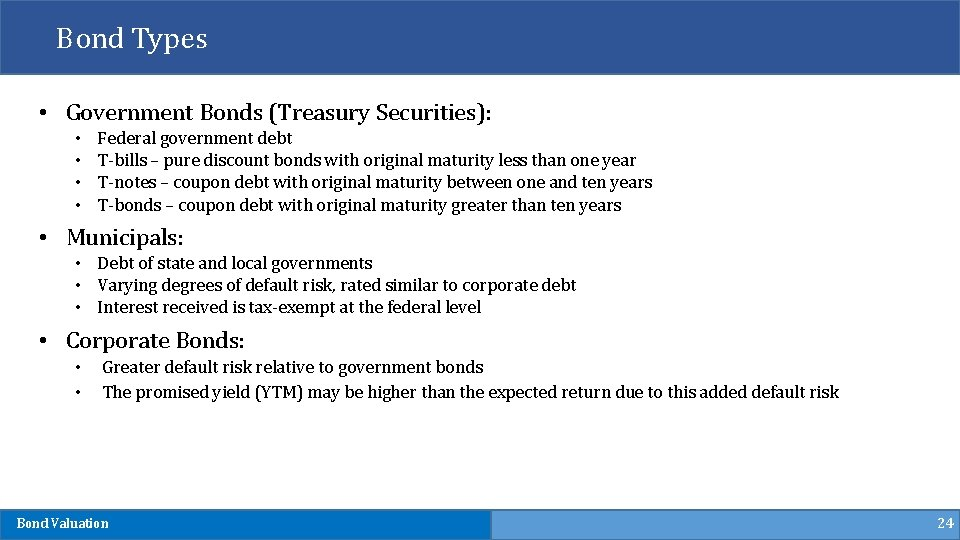Bond Types • Government Bonds (Treasury Securities): • • Federal government debt T-bills – pure discount bonds with original maturity less than one year T-notes – coupon debt with original maturity between one and ten years T-bonds – coupon debt with original maturity greater than ten years • Municipals: • Debt of state and local governments • Varying degrees of default risk, rated similar to corporate debt • Interest received is tax-exempt at the federal level • Corporate Bonds: • • Greater default risk relative to government bonds The promised yield (YTM) may be higher than the expected return due to this added default risk Bond Valuation 24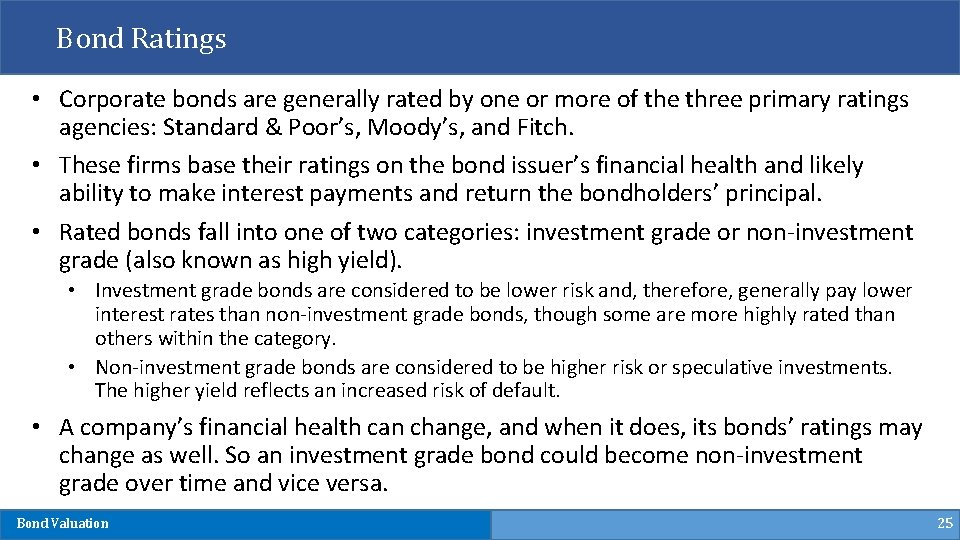Bond Ratings • Corporate bonds are generally rated by one or more of the three primary ratings agencies: Standard & Poor’s, Moody’s, and Fitch. • These firms base their ratings on the bond issuer’s financial health and likely ability to make interest payments and return the bondholders’ principal. • Rated bonds fall into one of two categories: investment grade or non-investment grade (also known as high yield). • Investment grade bonds are considered to be lower risk and, therefore, generally pay lower interest rates than non-investment grade bonds, though some are more highly rated than others within the category. • Non-investment grade bonds are considered to be higher risk or speculative investments. The higher yield reflects an increased risk of default. • A company’s financial health can change, and when it does, its bonds’ ratings may change as well. So an investment grade bond could become non-investment grade over time and vice versa. Bond Valuation 25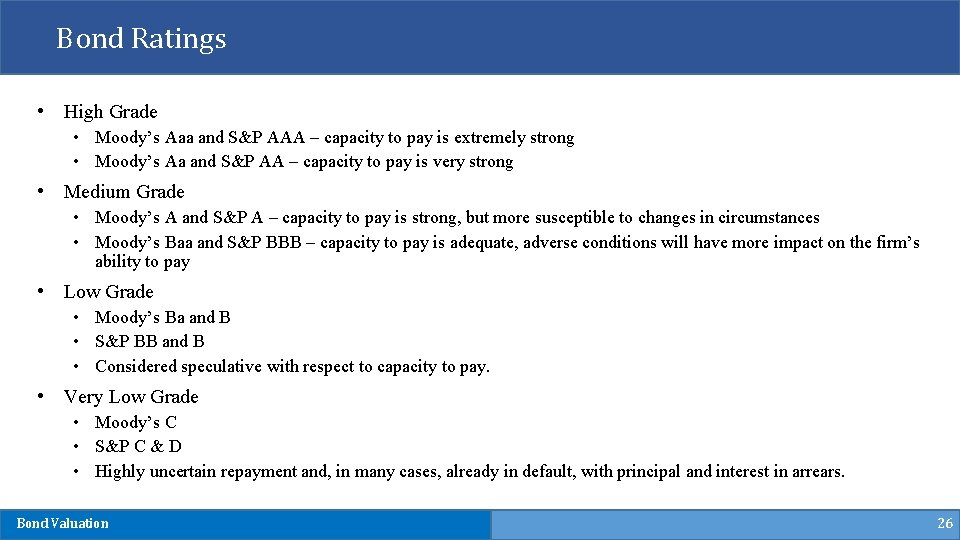Bond Ratings • High Grade • Moody’s Aaa and S&P AAA – capacity to pay is extremely strong • Moody’s Aa and S&P AA – capacity to pay is very strong • Medium Grade • Moody’s A and S&P A – capacity to pay is strong, but more susceptible to changes in circumstances • Moody’s Baa and S&P BBB – capacity to pay is adequate, adverse conditions will have more impact on the firm’s ability to pay • Low Grade • Moody’s Ba and B • S&P BB and B • Considered speculative with respect to capacity to pay. • Very Low Grade • Moody’s C • S&P C & D • Highly uncertain repayment and, in many cases, already in default, with principal and interest in arrears. Bond Valuation 26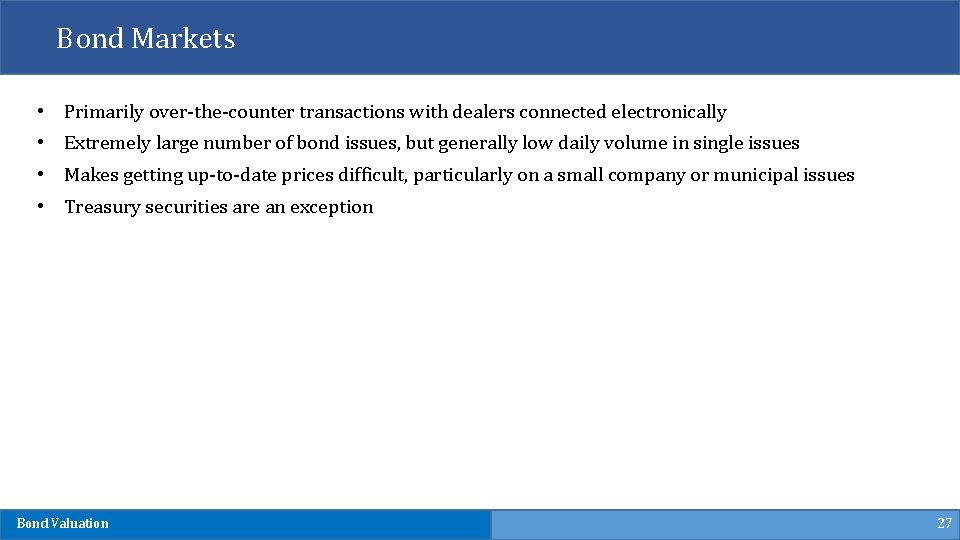Bond Markets • Primarily over-the-counter transactions with dealers connected electronically • Extremely large number of bond issues, but generally low daily volume in single issues • Makes getting up-to-date prices difficult, particularly on a small company or municipal issues • Treasury securities are an exception Bond Valuation 27Quick Summary- What does affect bond prices? • Interest Rate • Inflation: • • Inflationary conditions generally lead to a higher interest rate environment When the inflation rate rises, the price of a bond tends to drop, because the bond may not be paying enough interest to stay ahead of inflation. • Federal Fund Rate: • • The interest rate that financial institutions charge one another for short-term loans is called the federal funds rate. It’s determined by the U. S. Federal Reserve, which uses the federal funds rate as a lever to help balance the economy. • When the economy is slow, the Federal Reserve can lower the federal funds rate to encourage more borrowing, and when the economy is growing too fast, which can trigger large increases in inflation, the Federal Reserve can raise the federal funds rate to discourage borrowing. • Financial Health of Issuer (Default Risk) • Liquidity: • Bonds that have more frequent trading will generally have lower required returns Bond Valuation 28Interest Rate Risk • Price Risk • Change in price due to changes in interest rates • Long-term bonds have more price risk than short-term bonds • Low coupon rate bonds have more price risk than high coupon rate bonds. • Reinvestment Rate Risk • Uncertainty concerning rates at which cash flows can be reinvested • Short-term bonds have more reinvestment rate risk than long-term bonds. • High coupon rate bonds have more reinvestment rate risk than low coupon rate bonds. Bond Valuation 29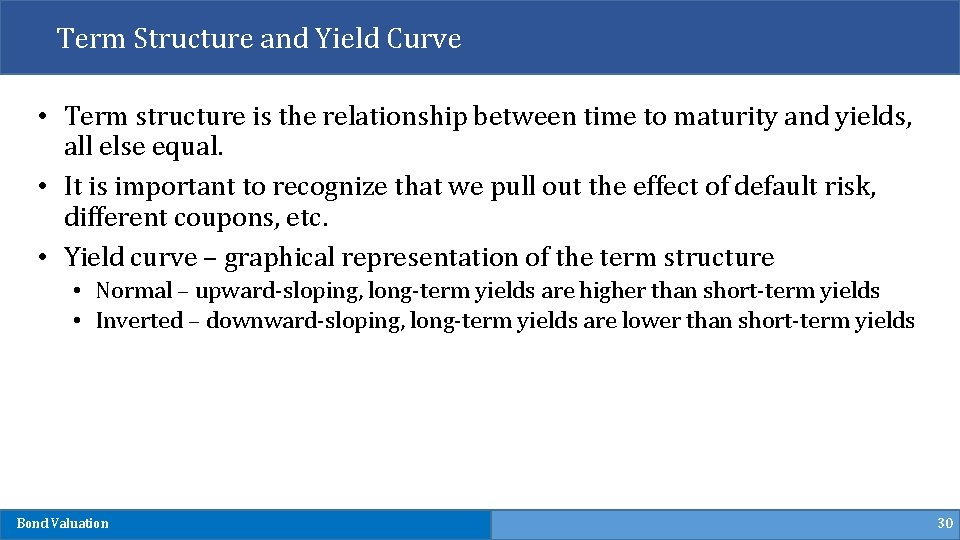Term Structure and Yield Curve • Term structure is the relationship between time to maturity and yields, all else equal. • It is important to recognize that we pull out the effect of default risk, different coupons, etc. • Yield curve – graphical representation of the term structure • Normal – upward-sloping, long-term yields are higher than short-term yields • Inverted – downward-sloping, long-term yields are lower than short-term yields Bond Valuation 30